krkr基础教程6

## 简单AVG元素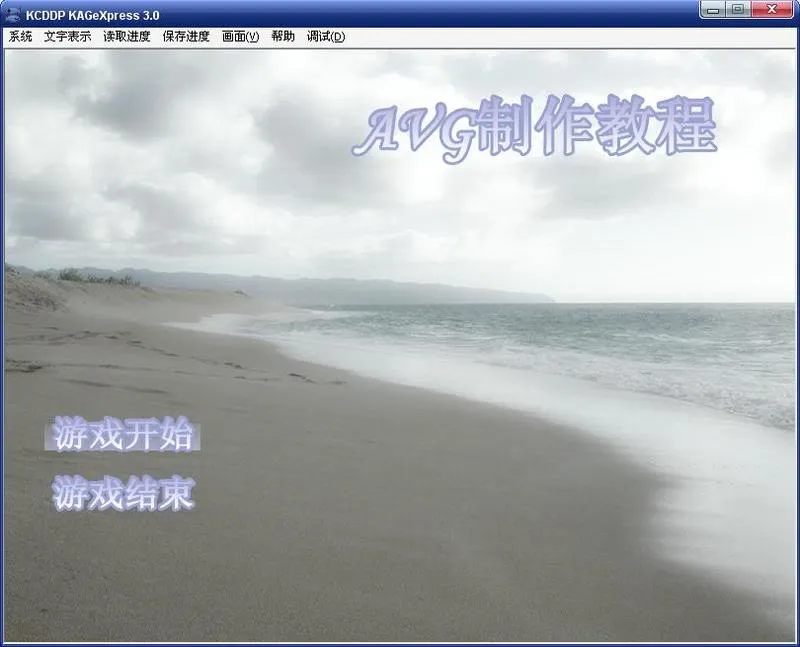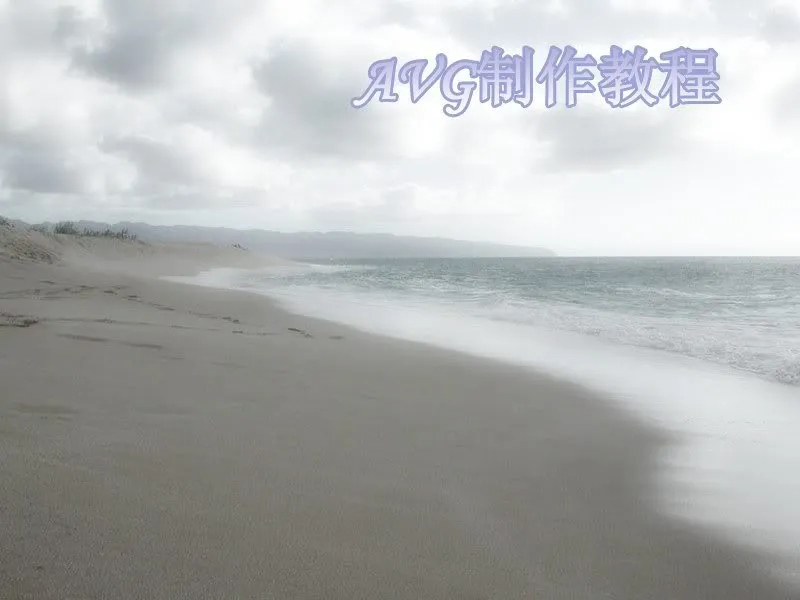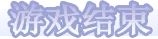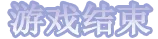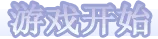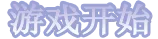*first
[rclick enabled=false]
;关闭右键
[clickskip enabled=false]
;关闭点击跳过
[history enabled=false output=false]
;关闭对话会放的开启和记录，不然按了鼠标滚轮就会开启对话回放
[playbgm storage="start"]
;这句是播放音乐，请随便在bgm文件夹里放一首你喜欢的音乐把它命名为start吧，注意根据音乐格式修改config.tjs里边的BGM_config，如果是mp3，ogg类的音乐就写type = "Wave"，因为mp3和ogg是用插件播放的，播放插件在func.ks里边已经进行载入了，这里可以直接使用

[position layer=message0 page=back visible=true opacity=0 top=0 height=600 left=0 width=800 marginl=0 margint=0 marginr=0 marginb=0 frame="opbg"]
[current layer=message0 page=back][er]
[locate x=40 y=370][button normal="opbt1" over="opbt1_1" storage="open.ks" target=*open]
;游戏开始按钮，点了游戏开始就跳转到open.ks这个脚本的*open标签开始执行
[locate x=40 y=430][button normal="opbt2" over="opbt2_1" exp="kag.onCloseQuery()"]
;kag.onCloseQuery()是一个tjs式，其实他是kag的一个函数，就是调用kag的关闭对话框，顺便一提，可能很多教程中都会忘记说明，kag这个东西，就是整个游戏窗口的名字，所有的图层，按钮，对话框，下拉菜单bulabulabula……都是在他的基础上建立的，具体他包含什么，可以查看mainwindow.tjs，大部分在做游戏时想不通的地方都可以去查那里……不过那东西太长了……我不建议大家逐行去看……单纯是什么地方想不通就查查那一处地方算了……
[s]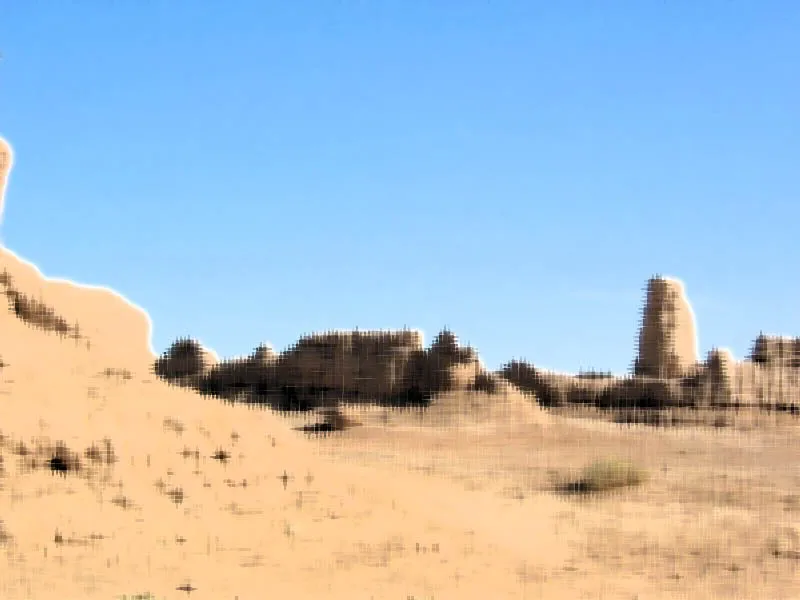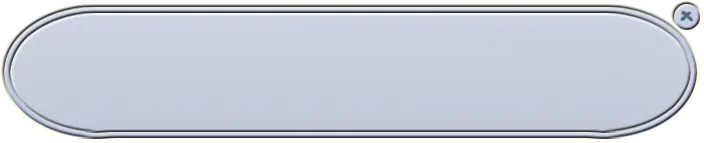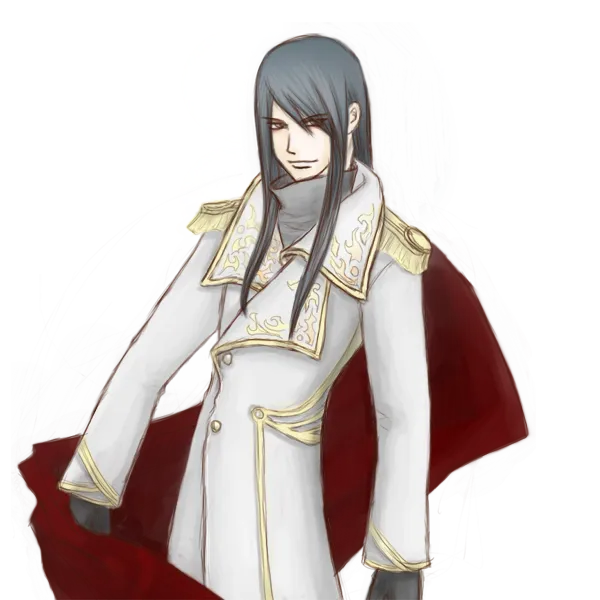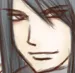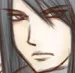*open|
[position layer=message0 page=back frame=""]
[current layer=message0 page=back][er]
;清空messge1，就是把封面清除掉

[playse storage="test"]
;这里就是演示一下播放音效的效果，请大家自己随便找一个wav格式的音乐文件放到sound文件夹里，改名为test

[image layer=base page=back storage="bg1" visible=true left=0 top=0 opacity=255]
;更改背景图片

[image layer=1 page=back storage="bt" visible=true left=100 top=0]
[pimage storage="btf2" layer=1 visible=true dx=260 dy=107 page=back]
;出现人物立绘

[position layer=message0 left=50 top=430 width=704 height=140 marginl=71 margint=17 marginr=73 marginb=17 opacity=0 visible=true frame="frame" page=back]
;出现对话框

[current layer=message0 pgae=fore]
[deffont face="黑体" size=20 color=0xffffff]
[defstyle linespacing=10 pitch=0 linesize=4]
[er]

;输出对话文字

[backlay]
[image layer=1 page=back storage="bt" visible=true left=100 top=0]
[pimage storage="btf1" layer=1 visible=true dx=260 dy=107 page=back]
;更改立绘

[current layer=message0 pgae=fore]

[position layer=message0 frame="" page=back]
;对话框消失

[freeimage layer=1 page=back]
;人物立绘消失

[freeimage layer=base page=back]
;背景消失

[jump target=*first storage="first.ks"]
;跳回开始画面

*open|
[position layer=message0 page=back frame=""]
[current layer=message0 page=back][er]
;清空messge1，就是把封面清除掉

@macro name=出现人物立绘
[backlay]
[image layer=1 page=back storage="bt" visible=true left=100 top=0]
[pimage storage=&("btf"+mp.face) layer=1 visible=true dx=260 dy=107 page=back cond="mp.face!=void"]
;mp变量相当于传递参数，只用在macro里边，在外边的代码里使用[出现人物立绘 face=1]，1这个值就会被传给mp.face，然后在macro里边进行使用，因为这个立绘有两张脸，我们在出立绘的时候只要写face的参数来控制角色用的哪个表情就可以了，同理也可以用这个来控制角色站立坐标，角色使用图层，trans时间之类之类的……
@endmacro

@macro name=出现对话框
[position layer=message0 left=50 top=430 width=704 height=140 marginl=71 margint=17 marginr=73 marginb=17 opacity=0 visible=true frame="frame" page=back]
@endmacro

@macro name=更改背景图片
[image layer=base page=back storage=%storage visible=true left=0 top=0 opacity=255]
;%这个符号也是代表后边的是传参，在这里写storage=%storage和storage=&mp.storage是等效的，这个也是只用在macro里的
@endmacro

@macro name=对话框消失
[position layer=message0 frame="" page=back]
@endmacro

@macro name=人物立绘消失
[freeimage layer=1 page=back]
@endmacro

@macro name=背景消失
[freeimage layer=base page=back]
@endmacro

[playse storage="test"]
;这里就是演示一下播放音效的效果，请大家自己随便找一个wav格式的音乐文件放到sound文件夹里，改名为test

[更改背景图片 storage="bg1"]
[出现人物立绘 face=2]
[出现对话框]

[current layer=message0 pgae=fore]
[deffont face="黑体" size=20 color=0xffffff]
[defstyle linespacing=10 pitch=0 linesize=4]
[er]

;输出对话文字

[出现人物立绘 face=1]
[current layer=message0 pgae=fore]

[对话框消失]
[人物立绘消失]
[背景消失]

[jump target=*first storage="first.ks"]
;跳回开始画面

clickskip
enabled

macro
name

endmacro

macro之后一定要有这个，不然后边你写的任何东西都算作这个macro里的，顺便一提，macro里不能有标签和tjs，不然会报错

erasemacro
name

history

output

enabled

playbgm

storage

loop

playse

storage

loop

buf

rclick
call

jump

call和jump这两个参数只能写一个
target

storage

enabled
false的话就是右键无效
name

aa